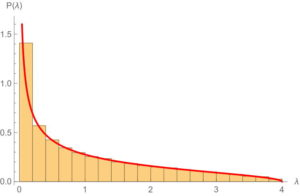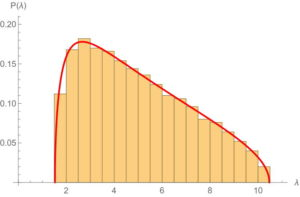# The joint distribution of marginals of multipartite random quantum states

Together with Stephane Dartois and Luca Lionni, we have a new preprint on the arXiv, On the joint distribution of the marginals of multipartite random quantum states. The paper is about the joint distribution of the set of (twisted) marginals of random multipartite quantum states. We study mainly random Wishart tensors, which, after normalization, yield multipartite random quantum states, so our work can also be seen as a contribution to the theory of random tensors.

Start with $2n$ copies of a finite dimensional complex Hilbert space, say $\mathbb C^n$. Consider $X \in (\mathbb C^N)^{\otimes 2n}$ a random complex Gaussian tensor, that is a vector of size $N^{2n}$ with i.i.d. standard complex Gaussian entries. for a subset $I \in \{1,2, \ldots, 2n\}$ of cardinality $n$, we define the marginal

$! \displaystyle W_{I} := [\mathrm{id}_I \otimes \operatorname{Tr}_{\hat I}](XX^*)$

obtained by partial tracing the tensor legs belonging to $\hat I$, the complementary set of $I$. Moreover, we allow for twisted marginals: for a permutation $\sigma \in \mathcal S_n$, let

$! \displaystyle W_{I, \sigma}:= P_\sigma^{-1} W_I P_\sigma,$

where $P_\sigma$ permutes the tensor legs as follows:

$! \displaystyle P_\sigma e_1 \otimes \cdots \otimes e_n = e_{\sigma(1)} \otimes \cdots \otimes e_{\sigma(n)}.$

We prove the following result.

Theorem 1 The set of ${{2n}\choose{n}} n!$ twisted marginals $W_{I,\sigma}$ are asymptotically free as $N \rightarrow \infty$. In other words, they jointly converge in distribution to a family of free standard free Poisson elements.

Recall that a free Poisson (or a Marchenko-Pastur) random variable has distribution

$! \displaystyle \mathrm{d}\mathrm{MP}_c=\max (1-c,0)\delta_0+\frac{\sqrt{(b-x)(x-a)}}{2\pi x} \; \mathbf{1}_{[a,b]}(x) \, \mathrm{d}x,$

where $c>0$ is a positive parameter (in our result, $c=1$). We display below the density (red curve) and numerical simulations for $c=1$and for $c=5$The message of our main theorem is that although the (overlapping) marginals are correlated random variables, their distributions become asymptotically free as the size of the individual Hilbert spaces grows to infinity.

The freeness result proven in the balanced asymptotical regime (the tensor factors scale in the same way) fails in the unbalanced asymptotical regime (some of the tensor factors have fixed dimensions, whereas others grow to infinity). We emphasize this fact in a particular situation, for $n=2$ (4-partite tensors). Write $W_{ABCD} = XX^*$, where

$! \displaystyle X \in \mathbb C^N \otimes \mathbb C^m \otimes \mathbb C^m \otimes \mathbb C^{\lfloor cN \rfloor},$

and $A,B,C,D$ denote, respectively, the four tensor factors above. It is natural to consider the joint distribution of the marginals $W_{AB},W_{AC}$, which are not asymptotically free anymore:

Theorem 2 As $N \rightarrow \infty$ and $c,m$ are fixed, the marginals $W_{AB},W_{AC}$ have the following asymptotical free cumulants:

$! \displaystyle \kappa(w_{f(1)}, w_{f(2)}, \ldots, w_{f(p)}) = cm^{-\mathrm{alt}(f)},$

where $f \in \{AB, AC\}^p$ is an arbitrary word in the letters $AB, AC$, and $\mathrm{alt}(f)$ is the number of different consecutive values of $f$, counted cyclically:

$! \displaystyle \mathrm{alt}(f) := |\{a \, : \, f(a) \neq f(a+1)\}|,$

where $f(p+1):=f(1)$.

The difference with the previous situation is that the amount of ”fresh” randomness in the marginals $W_{AB},W_{AC}$ is relatively small (fixed $m$ vs. $N \rightarrow \infty$), and not enough to ensure the asymptotical freeness. The natural framework to tackle such situations is that of freeness with amalgamation, and we shall study these cases in some future work.